# Principle Component Analysis PCA Networks 5 8 PCA

• Slides: 13Principle Component Analysis (PCA) Networks (§ 5. 8) • PCA: a statistical procedure – Reduce dimensionality of input vectors • Too many features, some of them are dependent of others • Extract important (new) features of data which are functions of original features • Minimize information loss in the process – This is done by forming new interesting features • As linear combinations of original features (first order of approximation) • New features are required to be linearly independent (to avoid redundancy) • New features are desired to be different from each other as much as possible (maximum variability)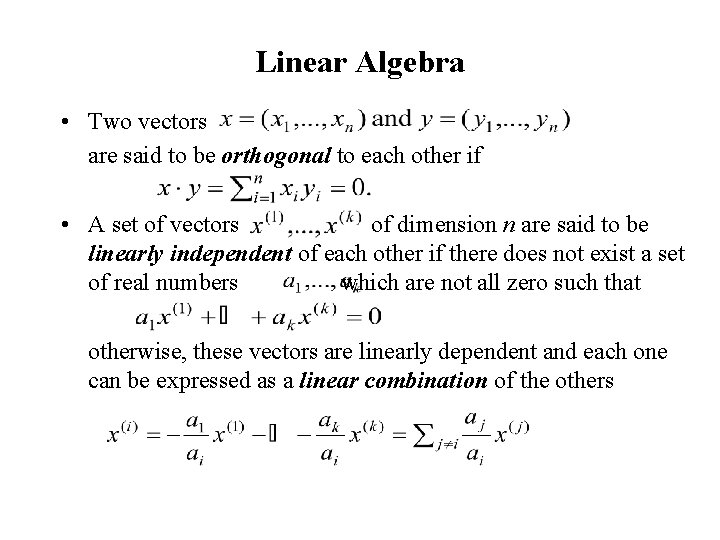Linear Algebra • Two vectors are said to be orthogonal to each other if • A set of vectors of dimension n are said to be linearly independent of each other if there does not exist a set of real numbers which are not all zero such that otherwise, these vectors are linearly dependent and each one can be expressed as a linear combination of the others• Vector x is an eigenvector of matrix A if there exists a constant != 0 such that Ax = x – is called a eigenvalue of A (wrt x) – A matrix A may have more than one eigenvectors, each with its own eigenvalue – Eigenvectors of a matrix corresponding to distinct eigenvalues are linearly independent of each other • Matrix B is called the inverse matrix of matrix A if AB = 1 – 1 is the identity matrix – Denote B as A-1 – Not every matrix has inverse (e. g. , when one of the row/column can be expressed as a linear combination of other rows/columns) • Every matrix A has a unique pseudo-inverse A*, which satisfies the following properties AA*A = A; A*AA* = A*; A*A = (A*A)T; AA* = (AA*)T• Example of PCA: 3 -dim x is transformed to 2 -dem y 2 -d feature vector Transformation 3 -d matrix W feature vector If rows of W have unit length and are orthogonal (e. g. , w 1 • w 2 = ap + bq + cr = 0), then WT is a pseudo-inverse of W• Generalization – Transform n-dim x to m-dem y (m < n) , the pseudo-inverse matrix W is a m x n matrix – Transformation: y = Wx – Opposite transformation: x’ = WTy = WTWx – If W minimizes “information loss” in the transformation, then ||x – x’|| = ||x – WTWx|| should also be minimized – If WT is the pseudo-inverse of W, then x’ = x: perfect transformation (no information loss) • How to find such a W for a given set of input vectors – Let T = {x 1, …, xk} be a set of input vectors – Making them zero-mean vectors by subtracting the mean vector (∑ xi) / k from each xi. – Compute the correlation matrix S(T) of these zero-mean vectors, which is a n x n matrix (book calls covariance-variance matrix)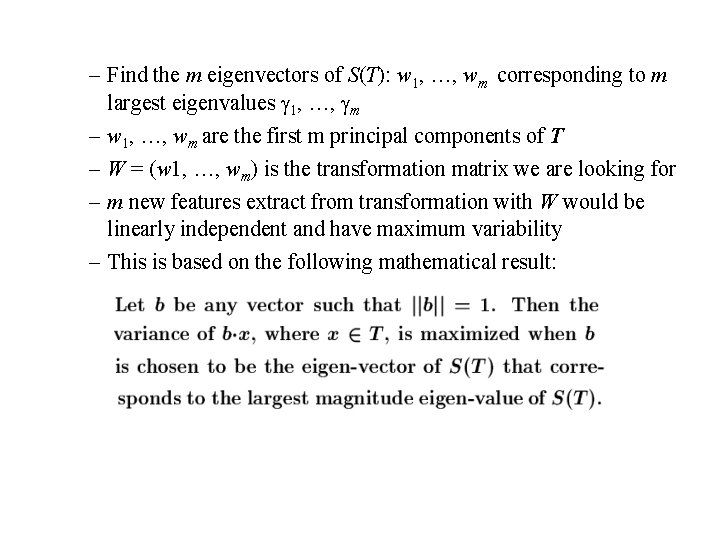– Find the m eigenvectors of S(T): w 1, …, wm corresponding to m largest eigenvalues 1, …, m – w 1, …, wm are the first m principal components of T – W = (w 1, …, wm) is the transformation matrix we are looking for – m new features extract from transformation with W would be linearly independent and have maximum variability – This is based on the following mathematical result: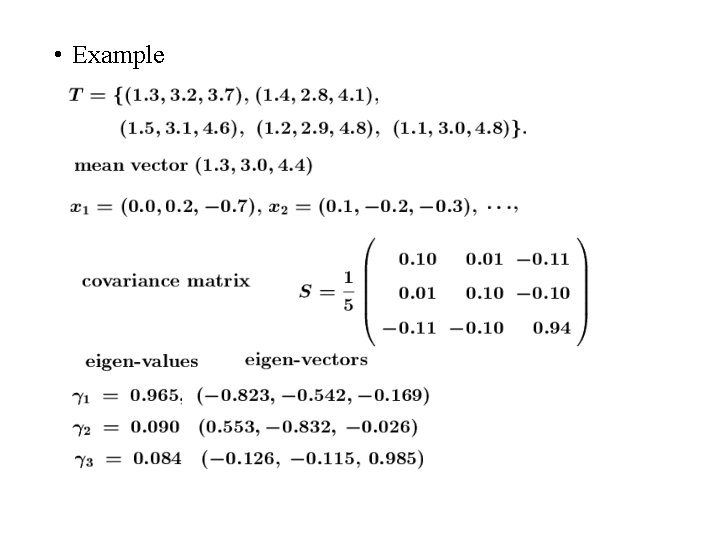• Example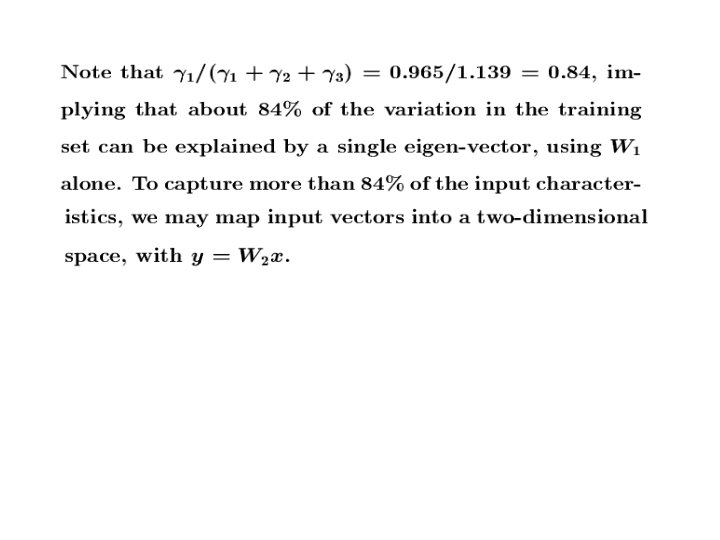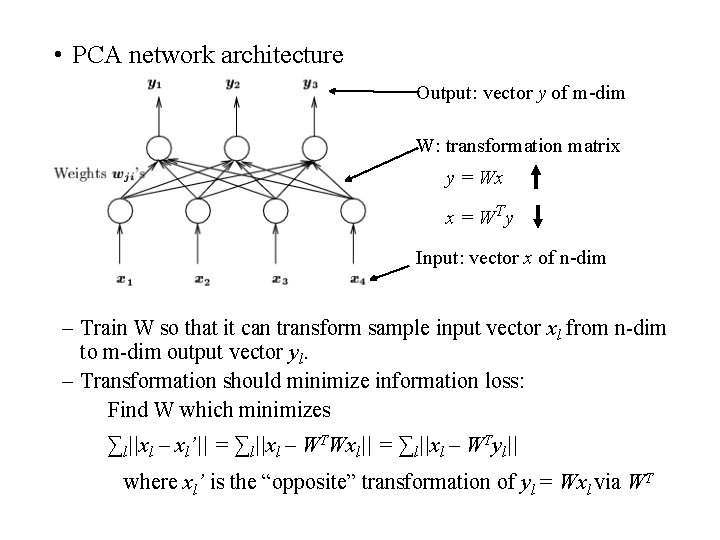• PCA network architecture Output: vector y of m-dim W: transformation matrix y = Wx x = WT y Input: vector x of n-dim – Train W so that it can transform sample input vector xl from n-dim to m-dim output vector yl. – Transformation should minimize information loss: Find W which minimizes ∑l||xl – xl’|| = ∑l||xl – WTWxl|| = ∑l||xl – WTyl|| where xl’ is the “opposite” transformation of yl = Wxl via WT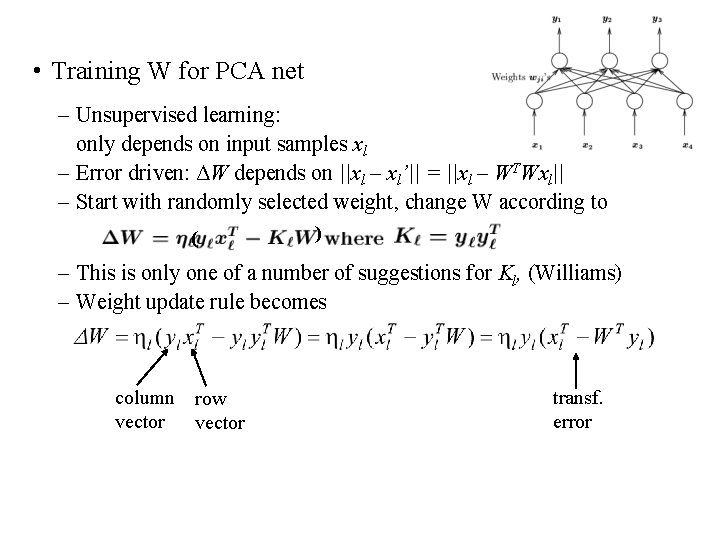• Training W for PCA net – Unsupervised learning: only depends on input samples xl – Error driven: ΔW depends on ||xl – xl’|| = ||xl – WTWxl|| – Start with randomly selected weight, change W according to ( ) – This is only one of a number of suggestions for Kl, (Williams) – Weight update rule becomes column vector row vector transf. error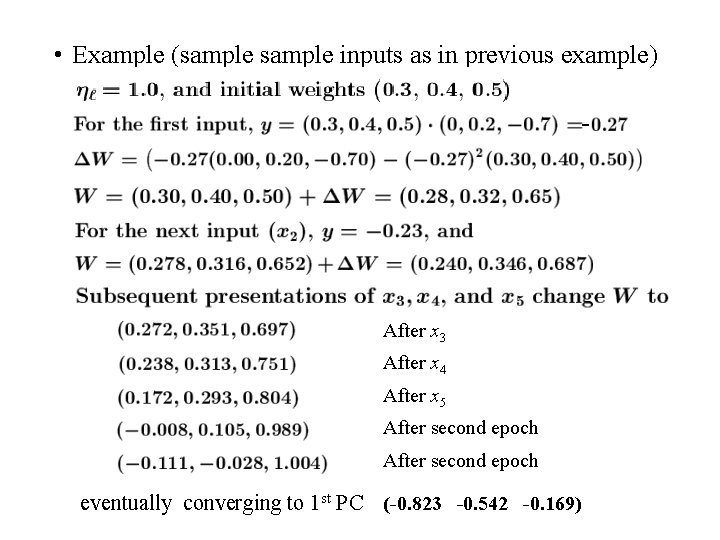• Example (sample inputs as in previous example) - After x 3 After x 4 After x 5 After second epoch eventually converging to 1 st PC (-0. 823 -0. 542 -0. 169)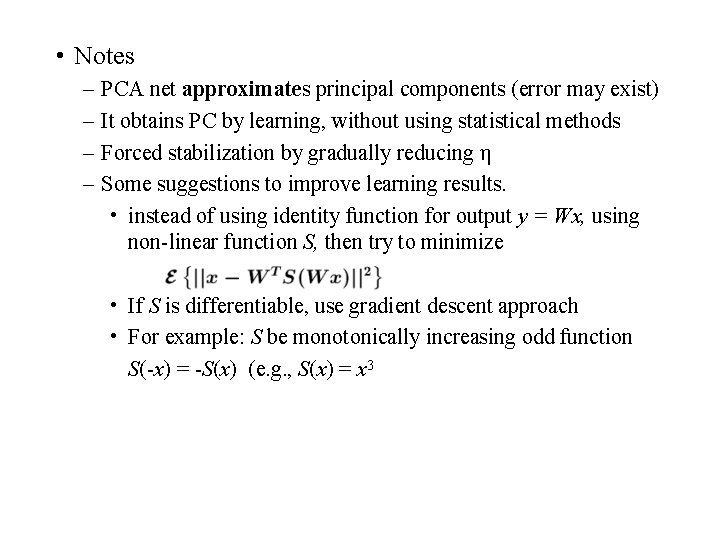• Notes – – PCA net approximates principal components (error may exist) It obtains PC by learning, without using statistical methods Forced stabilization by gradually reducing η Some suggestions to improve learning results. • instead of using identity function for output y = Wx, using non-linear function S, then try to minimize • If S is differentiable, use gradient descent approach • For example: S be monotonically increasing odd function S(-x) = -S(x) (e. g. , S(x) = x 3